# 11 Colt Aggregate Functions

This chapter provides a reference to Colt aggregate functions provided in Oracle Continuous Query Language (Oracle CQL). Colt aggregate functions are based on the Colt open source libraries for high performance scientific and technical computing.

This chapter includes the following section:

## 11.1 Introduction to Oracle CQL Built-In Aggregate Colt Functions

Table 11-1 lists the built-in aggregate Colt functions that Oracle CQL provides.

Table 11-1 Oracle CQL Built-in Aggregate Colt-Based Functions

Colt Package Function

`cern.jet.stat.Descriptive`

A set of basic descriptive statistics functions.

• `autoCorrelation`

• `correlation`

• `covariance`

• `geometricMean`

• `geometricMean1`

• `harmonicMean`

• `kurtosis`

• `lag1`

• `mean`

• `meanDeviation`

• `median`

• `moment`

• `pooledMean`

• `pooledVariance`

• `product`

• `quantile`

• `quantileInverse`

• `rankInterpolated`

• `rms`

• `sampleKurtosis`

• `sampleKurtosisStandardError`

• `sampleSkew`

• `sampleSkewStandardError`

• `sampleVariance`

• `skew`

• `standardDeviation`

• `standardError`

• `sumOfInversions`

• `sumOfLogarithms`

• `sumOfPowerDeviations`

• `sumOfPowers`

• `sumOfSquaredDeviations`

• `sumOfSquares`

• `trimmedMean`

• `variance`

• `weightedMean`

• `winsorizedMean`

Note:

Built-in function names are case sensitive and you must use them in the case shown (in lower case).

Note:

In stream input examples, lines beginning with `h` (such as `h 3800`) are heartbeat input tuples. These inform Oracle Event Processing that no further input will have a timestamp lesser than the heartbeat value.

In relation output examples, the first tuple output is:

```-9223372036854775808:+
```

This value is `-Long.MIN_VALUE()` and represents the largest negative timestamp possible.

### 11.1.1 Oracle CQL Colt Aggregate Function Signatures and Tuple Arguments

Note that the signatures of the Oracle CQL Colt aggregate functions do not match the signatures of the corresponding Colt aggregate functions.

Consider the following Colt aggregate function:

```double autoCorrelation(DoubleArrayList data, int lag, double mean, double variance)
```

In this signature, `data` is the `Collection` over which aggregates will be calculated and `mean` and `variance` are the other two parameter aggregates which are required to calculate `autoCorrelation` (where `mean` and `variance` aggregates are calculated on `data`).

In Oracle Event Processing, `data` will never come in the form of a `Collection`. The Oracle CQL function receives input data in a stream of tuples.

So suppose our stream is defined as `S:(double val, integer lag)`. On each input tuple, the Oracle CQL `autoCorrelation` function will compute two intermediate aggregates, `mean` and `variance`, and one final aggregate, `autoCorrelation`.

Since the function expects a stream of tuples having a `double` `data` value and an `integer` `lag` value only, the signature of the Oracle CQL `autoCorrelation` function is:

```double autoCorrelation (double data, int lag)
```

### 11.1.2 Colt Aggregate Functions and the Where, Group By, and Having Clauses

In Oracle CQL, the `where` clause is applied before the `group by` and `having` clauses. This means the Oracle CQL statement in Example 11-1 is invalid:

Example 11-1 Invalid Use of count

```<query id="q1"><![CDATA[
select * from InputChannel[rows 4 slide 4] as ic where geometricMean(c3) > 4
]]></query>
```

Instead, you must use the Oracle CQL statement that Example 11-2 shows:

Example 11-2 Valid Use of count

```<query id="q1"><![CDATA[
select * from InputChannel[rows 4 slide 4] as ic, myGeoMean = geometricMean(c3) where myGeoMean > 4
]]></query>
```

## autoCorrelation

Syntax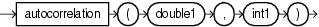Purpose

`autoCorrelation` is based on `cern.jet.stat.Descriptive.autoCorrelation(DoubleArrayList data, int lag, double mean, double variance)`. It returns the auto-correlation of a data sequence of the input arguments as a `double`.

Note:

This function has semantics different from "lag1"

This function takes the following tuple arguments:

• `double1`: data value.

• `int1`: lag.

Examples

Consider the query `qColtAggr1` in Example 11-3. Given the data stream `SColtAggrFunc` with schema `(c1 integer, c2 float, c3 double, c4 bigint)` in Example 11-4, the query returns the relation in Example 11-5.

Example 11-3 autoCorrelation Function Query

```<query id="qColtAggr1"><![CDATA[
select autoCorrelation(c3, 0) from SColtAggrFunc
]]></query>
```

Example 11-4 autoCorrelation Function Stream Input

```Timestamp   Tuple
10        5.441341838866902
1000        6.1593756700951054
1200        3.7269733222923676
1400        4.625160266213489
1600        3.490061774090248
1800        3.6354484064421917
2000        5.635401664977703
2200        5.006087562207967
2400        3.632574304861612
2600        7.618087248962962
h 8000
h 200000000
```

Example 11-5 autoCorrelation Function Relation Output

```Timestamp   Tuple Kind  Tuple
-9223372036854775808:+
10:       -
10:       +           NaN
1000:       -           NaN
1000:       +           1.0
1200:       -           1.0
1200:       +           1.0
1400:       -           1.0
1400:       +           1.0
1600:       -           1.0
1600:       +           1.000000000000002
1800:       -           1.000000000000002
1800:       +           1.0
2000:       -           1.0
2000:       +           0.9999999999999989
2200:       -           0.9999999999999989
2200:       +           0.999999999999999
2400:       -           0.999999999999999
2400:       +           0.9999999999999991
2600:       -           0.9999999999999991
2600:       +           1.0000000000000013
```

## correlation

Syntax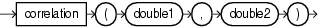Purpose

`correlation` is based on `cern.jet.stat.Descriptive.correlation(DoubleArrayList data1, double standardDev1, DoubleArrayList data2, double standardDev2)` . It returns the correlation of two data sequences of the input arguments as a `double`.

This function takes the following tuple arguments:

• `double1`: data value 1.

• `double2`: data value 2.

Examples

Consider the query `qColtAggr2` in Example 11-6. Given the data stream `SColtAggrFunc` with schema `(c1 integer, c2 float, c3 double, c4 bigint)` in Example 11-7, the query returns the relation in Example 11-8.

Example 11-6 correlation Function Query

```<query id="qColtAggr2"><![CDATA[
select correlation(c3, c3) from SColtAggrFunc
]]></query>
```

Example 11-7 correlation Function Stream Input

```Timestamp   Tuple
10        1, 0.5, 40.0, 8
1000        4, 0.7, 30.0, 6
1200        3, 0.89, 20.0, 12
2000        8, 0.4, 10.0, 4
h 8000
h 200000000
```

Example 11-8 correlation Function Relation Output

```Timestamp   Tuple Kind  Tuple
-9223372036854775808:+
10:       -
10:       +           NaN
1000:       -           NaN
1000:       +           2.0
1200:       -           2.0
1200:       +           1.5
2000:       -           1.5
2000:       +           1.333333333333333
```

## covariance

Syntax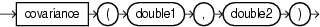Purpose

`covariance` is based on `cern.jet.stat.Descriptive.covariance(DoubleArrayList data1, DoubleArrayList data2)`. It returns the correlation of two data sequences (see Figure 11-1) of the input arguments as a `double`.

Figure 11-1 cern.jet.stat.Descriptive.covariance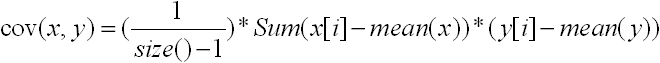This function takes the following tuple arguments:

• `double1`: data value 1.

• `double2`: data value 2.

Examples

Consider the query `qColtAggr3` in Example 11-9. Given the data stream `SColtAggrFunc` with schema `(c1 integer, c2 float, c3 double, c4 bigint)` in Example 11-10, the query returns the relation in Example 11-11.

Example 11-9 covariance Function Query

```<query id="qColtAggr3"><![CDATA[
select covariance(c3, c3) from SColtAggrFunc
]]></query>
```

Example 11-10 covariance Function Stream Input

```Timestamp   Tuple
10        1, 0.5, 40.0, 8
1000        4, 0.7, 30.0, 6
1200        3, 0.89, 20.0, 12
2000        8, 0.4, 10.0, 4
h 8000
h 200000000
```

Example 11-11 covariance Function Relation Output

```Timestamp   Tuple Kind  Tuple
-9223372036854775808:+
10:       -
10:       +           NaN
1000:       -           NaN
1000:       +           50.0
1200:       -           50.0
1200:       +           100.0
2000:       -           100.0
2000:       +           166.66666666666666
```

## geometricMean

SyntaxPurpose

`geometricMean` is based on `cern.jet.stat.Descriptive.geometricMean(DoubleArrayList data)`. It returns the geometric mean of a data sequence (see Figure 11-2) of the input argument as a `double`.

Figure 11-2 cern.jet.stat.Descriptive.geometricMean(DoubleArrayList data)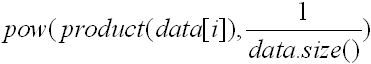This function takes the following tuple arguments:

• `double1`: data value.

Note that for a geometric mean to be meaningful, the minimum of the data values must not be less than or equal to zero.

Examples

Consider the query `qColtAggr6` in Example 11-12. Given the data stream `SColtAggrFunc` with schema `(c1 integer, c2 float, c3 double, c4 bigint)` in Example 11-13, the query returns the relation in Example 11-14.

Example 11-12 geometricMean Function Query

```<query id="qColtAggr6"><![CDATA[
select geometricMean(c3) from SColtAggrFunc
]]></query>
```

Example 11-13 geometricMean Function Stream Input

```Timestamp   Tuple
10        1, 0.5, 40.0, 8
1000        4, 0.7, 30.0, 6
1200        3, 0.89, 20.0, 12
2000        8, 0.4, 10.0, 4
h 8000
h 200000000
```

Example 11-14 geometricMean Function Relation Output

```Timestamp   Tuple Kind  Tuple
-9223372036854775808:+
10:       -
10:       +           40.0
1000:       -           40.0
1000:       +           34.64101615137755
1200:       -           34.64101615137755
1200:       +           28.844991406148168
2000:       -           28.844991406148168
2000:       +           22.133638394006436
```

## geometricMean1

Syntax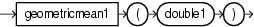Purpose

`geometricMean1` is based on `cern.jet.stat.Descriptive.geometricMean(double sumOfLogarithms)`. It returns the geometric mean of a data sequence (see Figure 11-3) of the input arguments as a `double`.

Figure 11-3 cern.jet.stat.Descriptive.geometricMean1(int size, double sumOfLogarithms)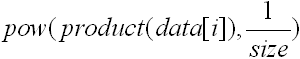This function takes the following tuple arguments:

• `double1`: data value.

Examples

Consider the query `qColtAggr7` in Example 11-15. Given the data stream `SColtAggrFunc` with schema `(c1 integer, c2 float, c3 double, c4 bigint)` in Example 11-16, the query returns the relation in Example 11-17.

Example 11-15 geometricMean1 Function Query

```<query id="qColtAggr7"><![CDATA[
select geometricMean1(c3) from SColtAggrFunc
]]></query>
```

Example 11-16 geometricMean1 Function Stream Input

```Timestamp   Tuple
10        1, 0.5, 40.0, 8
1000        4, 0.7, 30.0, 6
1200        3, 0.89, 20.0, 12
2000        8, 0.4, 10.0, 4
h 8000
h 200000000
```

Example 11-17 geometricMean1 Function Relation  Output

```Timestamp   Tuple Kind  Tuple
-9223372036854775808:+
10:       -
10:       +           Infinity
1000:       -           Infinity
1000:       +           Infinity
1200:       -           Infinity
1200:       +           Infinity
2000:       -           Infinity
2000:       +           Infinity
```

## harmonicMean

Syntax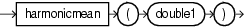Purpose

`harmonicMean` is based on `cern.jet.stat.Descriptive.harmonicMean(int size, double sumOfInversions)`. It returns the harmonic mean of a data sequence as a `double`.

This function takes the following tuple arguments:

• `double1`: data value.

Examples

Consider the query `qColtAggr8` in Example 11-18. Given the data stream `SColtAggrFunc` with schema `(c1 integer, c2 float, c3 double, c4 bigint)` in Example 11-19, the query returns the relation in Example 11-20.

Example 11-18 harmonicMean Function Query

```<query id="qColtAggr8"><![CDATA[
select harmonicMean(c3) from SColtAggrFunc
]]></query>
```

Example 11-19 harmonicMean Function Stream Input

```Timestamp   Tuple
10        5.441341838866902
1000        6.1593756700951054
1200        3.7269733222923676
1400        4.625160266213489
1600        3.490061774090248
1800        3.6354484064421917
2000        5.635401664977703
2200        5.006087562207967
2400        3.632574304861612
2600        7.618087248962962
h 8000
h 200000000
```

Example 11-20 harmonicMean Function Relation Output

```Timestamp   Tuple Kind  Tuple
-9223372036854775808:+
10:       -
10:       +           5.441341876983643
1000:       -           5.441341876983643
1000:       +           5.778137193205395
1200:       -           5.778137193205395
1200:       +           4.882442561720335
1400:       -           4.882442561720335
1400:       +           4.815475325819701
1600:       -           4.815475325819701
1600:       +           4.475541862878903
1800:       -           4.475541862878903
1800:       +           4.309563447664887
2000:       -           4.309563447664887
2000:       +           4.45944509362759
2200:       -           4.45944509362759
2200:       +           4.5211563834502515
2400:       -           4.5211563834502515
2400:       +           4.401525382790638
2600:       -           4.401525382790638
2600:       +           4.595562422157167
```

## kurtosis

Syntax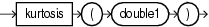Purpose

`kurtosis` is based on `cern.jet.stat.Descriptive.kurtosis(DoubleArrayList data, double mean, double standardDeviation)`. It returns the kurtosis or excess (see Figure 11-4) of a data sequence as a `double`.

Figure 11-4 cern.jet.stat.Descriptive.kurtosis(DoubleArrayList data, double mean, double standardDeviation)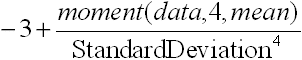This function takes the following tuple arguments:

• `double1`: data value.

Examples

Consider the query `qColtAggr12` in Example 11-21. Given the data stream `SColtAggrFunc` with schema `(c1 integer, c2 float, c3 double, c4 bigint)` in Example 11-22, the query returns the relation in Example 11-23.

Example 11-21 kurtosis Function Query

```<query id="qColtAggr12"><![CDATA[
select kurtosis(c3) from SColtAggrFunc
]]></query>
```

Example 11-22 kurtosis Function Stream Input

```Timestamp   Tuple
10        1, 0.5, 40.0, 8
1000        4, 0.7, 30.0, 6
1200        3, 0.89, 20.0, 12
2000        8, 0.4, 10.0, 4
h 8000
h 200000000
```

Example 11-23 kurtosis Function Relation Output

```Timestamp   Tuple Kind  Tuple
-9223372036854775808:+
10:       -
10:       +           NaN
1000:       -           NaN
1000:       +           -2.0
1200:       -           -2.0
1200:       +           -1.5000000000000002
2000:       -           -1.5000000000000002
2000:       +           -1.3600000000000003
```

## lag1

Syntax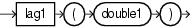Purpose

`lag1` is based on `cern.jet.stat.Descriptive.lag1(DoubleArrayList data, double mean)`. It returns the `lag - 1` auto-correlation of a dataset as a `double`.

Note:

This function has semantics different from "autoCorrelation".

This function takes the following tuple arguments:

• `double1`: data value.

Examples

Consider the query `qColtAggr14` in Example 11-24. Given the data stream `SColtAggrFunc` with schema `(c1 integer, c2 float, c3 double, c4 bigint)` in Example 11-25, the query returns the relation in Example 11-26.

Example 11-24 lag1 Function Query

```<query id="qColtAggr14"><![CDATA[
select lag1(c3) from SColtAggrFunc
]]></query>
```

Example 11-25 lag1 Function Stream Input

```Timestamp   Tuple
10        1, 0.5, 40.0, 8
1000        4, 0.7, 30.0, 6
1200        3, 0.89, 20.0, 12
2000        8, 0.4, 10.0, 4
h 8000
h 200000000
```

Example 11-26 lag1 Function Relation Output

```Timestamp   Tuple Kind  Tuple
-9223372036854775808:+
10:       -
10:       +           NaN
1000:       -           NaN
1000:       +           -0.5
1200:       -           -0.5
1200:       +           0.0
2000:       -           0.0
2000:       +           0.25
```

## mean

Syntax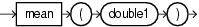Purpose

`mean` is based on `cern.jet.stat.Descriptive.mean(DoubleArrayList data)`. It returns the arithmetic mean of a data sequence (see Figure 11-5) as a `double`.

Figure 11-5 cern.jet.stat.Descriptive.mean(DoubleArrayList data)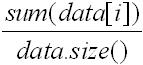This function takes the following tuple arguments:

• `double1`: data value.

Examples

Consider the query `qColtAggr16` in Example 11-27. Given the data stream `SColtAggrFunc` with schema `(c1 integer, c2 float, c3 double, c4 bigint)` in Example 11-28, the query returns the relation in Example 11-29.

Example 11-27 mean Function Query

```<query id="qColtAggr16"><![CDATA[
select mean(c3) from SColtAggrFunc
]]></query>
```

Example 11-28 mean Function Stream Input

```Timestamp   Tuple
10        1, 0.5, 40.0, 8
1000        4, 0.7, 30.0, 6
1200        3, 0.89, 20.0, 12
2000        8, 0.4, 10.0, 4
h 8000
h 200000000
```

Example 11-29 mean Function Relation Output

```Timestamp   Tuple Kind  Tuple
-9223372036854775808:+
10:       -
10:       +           40.0
1000:       -           40.0
1000:       +           35.0
1200:       -           35.0
1200:       +           30.0
2000:       -           30.0
2000:       +           25.0
```

## meanDeviation

Syntax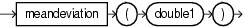Purpose

`meanDeviation` is based on `cern.jet.stat.Descriptive.meanDeviation(DoubleArrayList data, double mean)`. It returns the mean deviation of a dataset (see Figure 11-6) as a `double`.

Figure 11-6 cern.jet.stat.Descriptive.meanDeviation(DoubleArrayList data, double mean)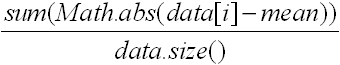This function takes the following tuple arguments:

• `double1`: data value.

Examples

Consider the query `qColtAggr17` in Example 11-30. Given the data stream `SColtAggrFunc` with schema `(c1 integer, c2 float, c3 double, c4 bigint)` in Example 11-31, the query returns the relation in Example 11-32.

Example 11-30 meanDeviation Function Query

```<query id="qColtAggr17"><![CDATA[
select meanDeviation(c3) from SColtAggrFunc
]]></query>
```

Example 11-31 meanDeviation Function Stream Input

```Timestamp   Tuple
10        1, 0.5, 40.0, 8
1000        4, 0.7, 30.0, 6
1200        3, 0.89, 20.0, 12
2000        8, 0.4, 10.0, 4
h 8000
h 200000000
```

Example 11-32 meanDeviation Function Relation Output

```Timestamp   Tuple Kind  Tuple
-9223372036854775808:+
10:       -
10:       +           0.0
1000:       -           0.0
1000:       +           5.0
1200:       -           5.0
1200:       +           6.666666666666667
2000:       -           6.666666666666667
2000:       +           10.0
```

## median

Syntax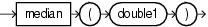Purpose

`median` is based on `cern.jet.stat.Descriptive.median(DoubleArrayList sortedData)`. It returns the median of a sorted data sequence as a `double`.

This function takes the following tuple arguments:

• `double1`: data value.

Examples

Consider the query `qColtAggr18` in Example 11-33. Given the data stream `SColtAggrFunc` with schema `(c1 integer, c2 float, c3 double, c4 bigint)` in Example 11-34, the query returns the relation in Example 11-35.

Example 11-33 median Function Query

```<query id="qColtAggr18"><![CDATA[
select median(c3) from SColtAggrFunc
]]></query>
```

Example 11-34 median Function Stream Input

```Timestamp   Tuple
10        1, 0.5, 40.0, 8
1000        4, 0.7, 30.0, 6
1200        3, 0.89, 20.0, 12
2000        8, 0.4, 10.0, 4
h 8000
h 200000000
```

Example 11-35 median Function Relation Output

```Timestamp   Tuple Kind  Tuple
-9223372036854775808:+
10:       -
10:       +           40.0
1000:       -           40.0
1000:       +           35.0
1200:       -           35.0
1200:       +           30.0
2000:       -           30.0
2000:       +           25.0
```

## moment

Syntax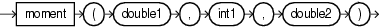Purpose

`moment` is based on `cern.jet.stat.Descriptive.moment(DoubleArrayList data, int k, double c)`. It returns the moment of the `k`-th order with constant `c` of a data sequence (see Figure 11-7) as a `double`.

Figure 11-7 cern.jet.stat.Descriptive.moment(DoubleArrayList data, int k, double c)This function takes the following tuple arguments:

• `double1`: data value.

• `int1`: `k`.

• `double2`: `c`.

Examples

Consider the query `qColtAggr21` in Example 11-36. Given the data stream `SColtAggrFunc` with schema `(c1 integer, c2 float, c3 double, c4 bigint)` in Example 11-37, the query returns the relation in Example 11-38.

Example 11-36 moment Function Query

```<query id="qColtAggr21"><![CDATA[
select moment(c3, c1, c3) from SColtAggrFunc
]]></query>
```

Example 11-37 moment Function Stream Input

```Timestamp   Tuple
10        1, 0.5, 40.0, 8
1000        4, 0.7, 30.0, 6
1200        3, 0.89, 20.0, 12
2000        8, 0.4, 10.0, 4
h 8000
h 200000000
```

Example 11-38 moment Function Relation Output

```Timestamp   Tuple Kind  Tuple
-9223372036854775808:+
10:       -
10:       +           0.0
1000:       -           0.0
1000:       +           5000.0
1200:       -           5000.0
1200:       +           3000.0
2000:       -           3000.0
2000:       +           1.7045E11
```

## pooledMean

Syntax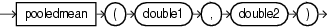Purpose

`pooledMean` is based on `cern.jet.stat.Descriptive.pooledMean(int size1, double mean1, int size2, double mean2)`. It returns the pooled mean of two data sequences (see Figure 11-8) as a `double`.

Figure 11-8 cern.jet.stat.Descriptive.pooledMean(int size1, double mean1, int size2, double mean2)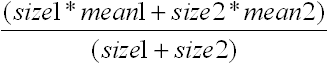This function takes the following tuple arguments:

• `double1`: mean 1.

• `double2`: mean 2.

Examples

Consider the query `qColtAggr22` in Example 11-39. Given the data stream `SColtAggrFunc` with schema `(c1 integer, c2 float, c3 double, c4 bigint)` in Example 11-40, the query returns the relation in Example 11-41.

Example 11-39 pooledMean Function Query

```<query id="qColtAggr22"><![CDATA[
select pooledMean(c3, c3) from SColtAggrFunc
]]></query>
```

Example 11-40 pooledMean Function Stream Input

```Timestamp   Tuple
10        1, 0.5, 40.0, 8
1000        4, 0.7, 30.0, 6
1200        3, 0.89, 20.0, 12
2000        8, 0.4, 10.0, 4
h 8000
h 200000000
```

Example 11-41 pooledMean Function Relation Output

```Timestamp   Tuple Kind  Tuple
-9223372036854775808:+
10:       -
10:       +           40.0
1000:       -           40.0
1000:       +           35.0
1200:       -           35.0
1200:       +           30.0
2000:       -           30.0
2000:       +           25.0
```

## pooledVariance

Syntax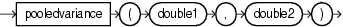Purpose

`pooledVariance` is based on `cern.jet.stat.Descriptive.pooledVariance(int size1, double variance1, int size2, double variance2)`. It returns the pooled variance of two data sequences (see Figure 11-9) as a `double`.

Figure 11-9 cern.jet.stat.Descriptive.pooledVariance(int size1, double variance1, int size2, double variance2)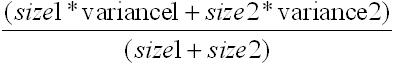This function takes the following tuple arguments:

• `double1`: variance 1.

• `double2`: variance 2.

Examples

Consider the query `qColtAggr23` in Example 11-42. Given the data stream `SColtAggrFunc` with schema `(c1 integer, c2 float, c3 double, c4 bigint)` in Example 11-43, the query returns the relation in Example 11-44.

Example 11-42 pooledVariance Function Query

```<query id="qColtAggr23"><![CDATA[
select pooledVariance(c3, c3) from SColtAggrFunc
]]></query>
```

Example 11-43 pooledVariance Function Stream Input

```Timestamp   Tuple
10        1, 0.5, 40.0, 8
1000        4, 0.7, 30.0, 6
1200        3, 0.89, 20.0, 12
2000        8, 0.4, 10.0, 4
h 8000
h 200000000
```

Example 11-44 pooledVariance Function Relation Output

```Timestamp   Tuple Kind  Tuple
-9223372036854775808:+
10:       -
10:       +           0.0
1000:       -           0.0
1000:       +           25.0
1200:       -           25.0
1200:       +           66.66666666666667
2000:       -           66.66666666666667
2000:       +           125.0
```

## product

Syntax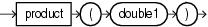Purpose

`product` is based on `cern.jet.stat.Descriptive.product(DoubleArrayList data)`. It returns the product of a data sequence (see Figure 11-10) as a `double`.

Figure 11-10 cern.jet.stat.Descriptive.product(DoubleArrayList data)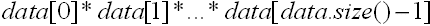This function takes the following tuple arguments:

• `double1`: data value.

Examples

Consider the query `qColtAggr24` in Example 11-45. Given the data stream `SColtAggrFunc` with schema `(c1 integer, c2 float, c3 double, c4 bigint)` in Example 11-46, the query returns the relation in Example 11-47.

Example 11-45 product Function Query

```<query id="qColtAggr24"><![CDATA[
select product(c3) from SColtAggrFunc
]]></query>
```

Example 11-46 product Function Stream Input

```Timestamp   Tuple
10        1, 0.5, 40.0, 8
1000        4, 0.7, 30.0, 6
1200        3, 0.89, 20.0, 12
2000        8, 0.4, 10.0, 4
h 8000
h 200000000
```

Example 11-47 product Function Relation Output

```Timestamp   Tuple Kind  Tuple
-9223372036854775808:+
10:       -
10:       +           40.0
1000:       -           40.0
1000:       +           1200.0
1200:       -           1200.0
1200:       +           24000.0
2000:       -           24000.0
2000:       +           240000.0
```

## quantile

Syntax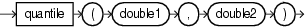Purpose

`quantile` is based on `cern.jet.stat.Descriptive.quantile(DoubleArrayList sortedData, double phi)`. It returns the phi-quantile as a `double`; that is, an element `elem` for which holds that phi percent of data elements are less than `elem`.

This function takes the following tuple arguments:

• `double1`: data value.

• `double2`: phi; the percentage; must satisfy `0 <= phi <= 1`.

Examples

Consider the query `qColtAggr26` in Example 11-48. Given the data stream `SColtAggrFunc` with schema `(c1 integer, c2 float, c3 double, c4 bigint)` in Example 11-49, the query returns the relation in Example 11-50.

Example 11-48 quantile Function Query

```<query id="qColtAggr26"><![CDATA[
select quantile(c3, c2) from SColtAggrFunc
]]></query>
```

Example 11-49 quantile Function Stream Input

```Timestamp   Tuple
10        1, 0.5, 40.0, 8
1000        4, 0.7, 30.0, 6
1200        3, 0.89, 20.0, 12
2000        8, 0.4, 10.0, 4
h 8000
h 200000000
```

Example 11-50 quantile Function Relation Output

```Timestamp   Tuple Kind  Tuple
-9223372036854775808:+
10:         -
10:         +         40.0
1000:         -         40.0
1000:         +         36.99999988079071
1200:         -         36.99999988079071
1200:         +         37.799999713897705
2000:         -         37.799999713897705
2000:         +         22.000000178813934
```

## quantileInverse

Syntax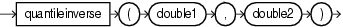Purpose

`quantileInverse` is based on `cern.jet.stat.Descriptive.quantileInverse(DoubleArrayList sortedList, double element)`. It returns the percentage phi of elements `<= element` (`0.0 <= phi <= 1.0`) as a `double`. This function does linear interpolation if the `element` is not contained but lies in between two contained elements.

This function takes the following tuple arguments:

• `double1`: data.

• `double2`: `element`.

Examples

Consider the query `qColtAggr27` in Example 11-51. Given the data stream `SColtAggrFunc` with schema `(c1 integer, c2 float, c3 double, c4 bigint)` in Example 11-52, the query returns the relation in Example 11-53.

Example 11-51 quantileInverse Function Query

```<query id="qColtAggr27"><![CDATA[
select quantileInverse(c3, c3) from SColtAggrFunc
]]></query>
```

Example 11-52 quantileInverse Function Stream Input

```Timestamp   Tuple
10        1, 0.5, 40.0, 8
1000        4, 0.7, 30.0, 6
1200        3, 0.89, 20.0, 12
2000        8, 0.4, 10.0, 4
h 8000
h 200000000
```

Example 11-53 quantileInverse Function Relation Output

```Timestamp   Tuple Kind  Tuple
-9223372036854775808:+
10:       -
10:       +           1.0
1000:       -           1.0
1000:       +           0.5
1200:       -           0.5
1200:       +           0.3333333333333333
2000:       -           0.3333333333333333
2000:       +           0.25
```

## rankInterpolated

Syntax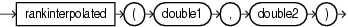Purpose

`rankInterpolated` is based on `cern.jet.stat.Descriptive.rankInterpolated(DoubleArrayList sortedList, double element)`. It returns the linearly interpolated number of elements in a list less or equal to a given `element` as a double.

The rank is the number of elements `<= element`. Ranks are of the form`{0, 1, 2,..., sortedList.size()}`. If no element is `<= element`, then the rank is zero. If the element lies in between two contained elements, then linear interpolation is used and a non-integer value is returned.

This function takes the following tuple arguments:

• `double1`: data value.

• `double2`: `element`.

Examples

Consider the query `qColtAggr29` in Example 11-54. Given the data stream `SColtAggrFunc` with schema `(c1 integer, c2 float, c3 double, c4 bigint)` in Example 11-55, the query returns the relation in Example 11-56.

Example 11-54 rankInterpolated Function Query

```<query id="qColtAggr29"><![CDATA[
select rankInterpolated(c3, c3) from SColtAggrFunc
]]></query>
```

Example 11-55 rankInterpolated Function Stream Input

```Timestamp   Tuple
10        1, 0.5, 40.0, 8
1000        4, 0.7, 30.0, 6
1200        3, 0.89, 20.0, 12
2000        8, 0.4, 10.0, 4
h 8000
h 200000000
```

Example 11-56 rankInterpolated Function Relation Output

```Timestamp   Tuple Kind  Tuple
-9223372036854775808:+
10:       -
10:       +           1.0
1000:       -           1.0
1000:       +           1.0
1200:       -           1.0
1200:       +           1.0
2000:       -           1.0
2000:       +           1.0
```

## rms

Syntax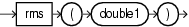Purpose

`rms` is based on `cern.jet.stat.Descriptive.rms(int size, double sumOfSquares)`. It returns the Root-Mean-Square (RMS) of a data sequence (see Figure 11-11) as a `double`.

Figure 11-11 cern.jet.stat.Descriptive.rms(int size, double sumOfSquares)This function takes the following tuple arguments:

• `double1`: data value.

Examples

Consider the query `qColtAggr30` in Example 11-57. Given the data stream `SColtAggrFunc` with schema `(c1 integer, c2 float, c3 double, c4 bigint)` in Example 11-58, the query returns the relation in Example 11-59.

Example 11-57 rms Function Query

```<query id="qColtAggr30"><![CDATA[
select rms(c3) from SColtAggrFunc
]]></query>
```

Example 11-58 rms Function Stream Input

```Timestamp   Tuple
10        1, 0.5, 40.0, 8
1000        4, 0.7, 30.0, 6
1200        3, 0.89, 20.0, 12
2000        8, 0.4, 10.0, 4
h 8000
h 200000000
```

Example 11-59 rms Function Relation Output

```Timestamp   Tuple Kind  Tuple
-9223372036854775808:+
10:       -
10:       +           40.0
1000:       -           40.0
1000:       +           35.35533905932738
1200:       -           35.35533905932738
1200:       +           31.09126351029605
2000:       -           31.09126351029605
2000:       +           27.386127875258307
```

## sampleKurtosis

Syntax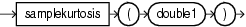Purpose

`sampleKurtosis` is based on `cern.jet.stat.Descriptive.sampleKurtosis(DoubleArrayList data, double mean, double sampleVariance)`. It returns the sample kurtosis (excess) of a data sequence as a `double`.

This function takes the following tuple arguments:

• `double1`: data value.

Examples

Consider the query `qColtAggr31` in Example 11-60. Given the data stream `SColtAggrFunc` with schema `(c1 integer, c2 float, c3 double, c4 bigint)` in Example 11-61, the query returns the relation in Example 11-62.

Example 11-60 sampleKurtosis Function Query

```<query id="qColtAggr31"><![CDATA[
select sampleKurtosis(c3) from SColtAggrFunc
]]></query>
```

Example 11-61 sampleKurtosis Function Stream Input

```Timestamp   Tuple
10        1, 0.5, 40.0, 8
1000        4, 0.7, 30.0, 6
1200        3, 0.89, 20.0, 12
2000        8, 0.4, 10.0, 4
h 8000
h 200000000
```

Example 11-62 sampleKurtosis Function Relation Output

```Timestamp   Tuple Kind  Tuple
-9223372036854775808:+
10:       -
10:       +           NaN
1000:       -           NaN
1000:       +           NaN
1200:       -           NaN
1200:       +           NaN
2000:       -           NaN
2000:       +           -1.1999999999999993
```

## sampleKurtosisStandardError

Syntax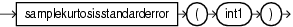Purpose

`sampleKurtosisStandardError` is based on `cern.jet.stat.Descriptive.sampleKurtosisStandardError(int size)`. It returns the standard error of the sample Kurtosis as a `double`.

This function takes the following tuple arguments:

• `int1`: data value.

Examples

Consider the query `qColtAggr33` in Example 11-63. Given the data stream `SColtAggrFunc` with schema `(c1 integer, c2 float, c3 double, c4 bigint)` in Example 11-64, the query returns the relation in Example 11-65.

Example 11-63 sampleKurtosisStandardError Function Query

```<query id="qColtAggr33"><![CDATA[
select sampleKurtosisStandardError(c1) from SColtAggrFunc
]]></query>
```

Example 11-64 sampleKurtosisStandardError Function Stream Input

```Timestamp   Tuple
10        1, 0.5, 40.0, 8
1000        4, 0.7, 30.0, 6
1200        3, 0.89, 20.0, 12
2000        8, 0.4, 10.0, 4
h 8000
h 200000000
```

Example 11-65 sampleKurtosisStandardError Function Relation Output

```Timestamp   Tuple Kind  Tuple
-9223372036854775808:+
10:       -
10:       +           0.0
1000:       -           0.0
1000:       +           Infinity
1200:       -           Infinity
1200:       +           Infinity
2000:       -           Infinity
2000:       +           2.6186146828319083
```

## sampleSkew

Syntax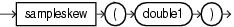Purpose

`sampleSkew` is based on `cern.jet.stat.Descriptive.sampleSkew(DoubleArrayList data, double mean, double sampleVariance)`. It returns the sample skew of a data sequence as a `double`.

This function takes the following tuple arguments:

• `double1`: data value.

Examples

Consider the query `qColtAggr34` in Example 11-66. Given the data stream `SColtAggrFunc` with schema `(c1 integer, c2 float, c3 double, c4 bigint)` in Example 11-67, the query returns the relation in Example 11-68.

Example 11-66 sampleSkew Function Query

```<query id="qColtAggr34"><![CDATA[
select sampleSkew(c3) from SColtAggrFunc
]]></query>
```

Example 11-67 sampleSkew Function Stream Input

```Timestamp   Tuple
10        1, 0.5, 40.0, 8
1000        4, 0.7, 30.0, 6
1200        3, 0.89, 20.0, 12
2000        8, 0.4, 10.0, 4
h 8000
h 200000000
```

Example 11-68 sampleSkew Function Relation Output

```Timestamp   Tuple Kind  Tuple
-9223372036854775808:+
10:       -
10:       +           NaN
1000:       -           NaN
1000:       +           NaN
1200:       -           NaN
1200:       +           0.0
2000:       -           0.0
2000:       +           0.0
```

## sampleSkewStandardError

Syntax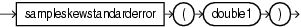Purpose

`sampleSkewStandardError` is based on `cern.jet.stat.Descriptive.sampleSkewStandardError(int size)`. It returns the standard error of the sample skew as a `double`.

This function takes the following tuple arguments:

• `double1`: data value.

Examples

Consider the query `qColtAggr36` in Example 11-69. Given the data stream `SColtAggrFunc` with schema `(c1 integer, c2 float, c3 double, c4 bigint)` in Example 11-70, the query returns the relation in Example 11-71.

Example 11-69 sampleSkewStandardError Function Query

```<query id="qColtAggr36"><![CDATA[
select sampleSkewStandardError(c1) from SColtAggrFunc
]]></query>
```

Example 11-70 sampleSkewStandardError Function Stream Input

```Timestamp   Tuple
10        1, 0.5, 40.0, 8
1000        4, 0.7, 30.0, 6
1200        3, 0.89, 20.0, 12
2000        8, 0.4, 10.0, 4
h 8000
h 200000000
```

Example 11-71 sampleSkewStandardError Function Relation Output

```Timestamp   Tuple Kind  Tuple
-9223372036854775808:+
10:       -
10:       +           -0.0
1000:       -           -0.0
1000:       +           Infinity
1200:       -           Infinity
1200:       +           1.224744871391589
2000:       -           1.224744871391589
2000:       +           1.01418510567422
```

## sampleVariance

Syntax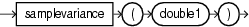Purpose

`sampleVariance` is based on `cern.jet.stat.Descriptive.sampleVariance(DoubleArrayList data, double mean)`. It returns the sample variance of a data sequence (see Figure 11-12) as a `double`.

Figure 11-12 cern.jet.stat.Descriptive.sampleVariance(DoubleArrayList data, double mean)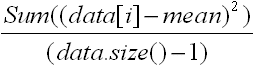This function takes the following tuple arguments:

• `double1`: data value.

Examples

Consider the query `qColtAggr38` in Example 11-72. Given the data stream `SColtAggrFunc` with schema `(c1 integer, c2 float, c3 double, c4 bigint)` in Example 11-73, the query returns the relation in Example 11-74.

Example 11-72 sampleVariance Function Query

```<query id="qColtAggr38"><![CDATA[
select sampleVariance(c3) from SColtAggrFunc
]]></query>
```

Example 11-73 sampleVariance Function Stream Input

```Timestamp   Tuple
10        1, 0.5, 40.0, 8
1000        4, 0.7, 30.0, 6
1200        3, 0.89, 20.0, 12
2000        8, 0.4, 10.0, 4
h 8000
h 200000000
```

Example 11-74 sampleVariance Function Relation Output

```Timestamp   Tuple Kind  Tuple
-9223372036854775808:+
10:       -
10:       +           NaN
1000:       -           NaN
1000:       +           50.0
1200:       -           50.0
1200:       +           100.0
2000:       -           100.0
2000:       +           166.66666666666666
```

## skew

Syntax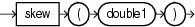Purpose

`skew` is based on `cern.jet.stat.Descriptive.skew(DoubleArrayList data, double mean, double standardDeviation)`. It returns the skew of a data sequence of a data sequence (see Figure 11-13) as a `double`.

Figure 11-13 cern.jet.stat.Descriptive.skew(DoubleArrayList data, double mean, double standardDeviation)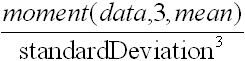This function takes the following tuple arguments:

• `double1`: data value.

Examples

Consider the query `qColtAggr41` in Example 11-75. Given the data stream `SColtAggrFunc` with schema `(c1 integer, c2 float, c3 double, c4 bigint)` in Example 11-76, the query returns the relation in Example 11-77.

Example 11-75 skew Function Query

```<query id="qColtAggr41"><![CDATA[
select skew(c3) from SColtAggrFunc
]]></query>
```

Example 11-76 skew Function Stream Input

```Timestamp   Tuple
10        1, 0.5, 40.0, 8
1000        4, 0.7, 30.0, 6
1200        3, 0.89, 20.0, 12
2000        8, 0.4, 10.0, 4
h 8000
h 200000000
```

Example 11-77 skew Function Relation Output

```Timestamp   Tuple Kind  Tuple
-9223372036854775808:+
10:       -
10:       +           NaN
1000:       -           NaN
1000:       +           0.0
1200:       -           0.0
1200:       +           0.0
2000:       -           0.0
2000:       +           0.0
```

## standardDeviation

Syntax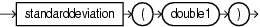Purpose

`standardDeviation` is based on `cern.jet.stat.Descriptive.standardDeviation(double variance)`. It returns the standard deviation from a variance as a `double`.

This function takes the following tuple arguments:

• `double1`: data value.

Examples

Consider the query `qColtAggr44` in Example 11-78. Given the data stream `SColtAggrFunc` with schema `(c1 integer, c2 float, c3 double, c4 bigint)` in Example 11-79, the query returns the relation in Example 11-80.

Example 11-78 standardDeviation Function Query

```<query id="qColtAggr44"><![CDATA[
select standardDeviation(c3) from SColtAggrFunc
]]></query>
```

Example 11-79 standardDeviation Function Stream Input

```Timestamp   Tuple
10        1, 0.5, 40.0, 8
1000        4, 0.7, 30.0, 6
1200        3, 0.89, 20.0, 12
2000        8, 0.4, 10.0, 4
h 8000
h 200000000
```

Example 11-80 standardDeviation Function Relation Output

```Timestamp   Tuple Kind  Tuple
-9223372036854775808:+
10:       -
10:       +           0.0
1000:       -           0.0
1000:       +           5.0
1200:       -           5.0
1200:       +           8.16496580927726
2000:       -           8.16496580927726
2000:       +           11.180339887498949
```

## standardError

Syntax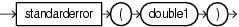Purpose

`standardError` is based on `cern.jet.stat.Descriptive.standardError(int size, double variance)`. It returns the standard error of a data sequence (see Figure 11-14) as a `double`.

Figure 11-14 cern.jet.stat.Descriptive.cern.jet.stat.Descriptive.standardError(int size, double variance)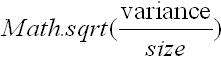This function takes the following tuple arguments:

• `double1`: data value.

Examples

Consider the query `qColtAggr45` in Example 11-81. Given the data stream `SColtAggrFunc` with schema `(c1 integer, c2 float, c3 double, c4 bigint)` in Example 11-82, the query returns the relation in Example 11-83.

Example 11-81 standardError Function Query

```<query id="qColtAggr45"><![CDATA[
select standardError(c3) from SColtAggrFunc
]]></query>
```

Example 11-82 standardError Function Stream Input

```Timestamp   Tuple
10        1, 0.5, 40.0, 8
1000        4, 0.7, 30.0, 6
1200        3, 0.89, 20.0, 12
2000        8, 0.4, 10.0, 4
h 8000
h 200000000
```

Example 11-83 standardError Function Relation Output

```Timestamp   Tuple Kind  Tuple
-9223372036854775808:+
10:       -
10:       +           0.0
1000:       -           0.0
1000:       +           3.5355339059327378
1200:       -           3.5355339059327378
1200:       +           4.714045207910317
2000:       -           4.714045207910317
2000:       +           5.5901699437494745
```

## sumOfInversions

Syntax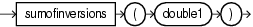Purpose

`sumOfInversions` is based on `cern.jet.stat.Descriptive.sumOfInversions(DoubleArrayList data, int from, int to)`. It returns the sum of inversions of a data sequence (see Figure 11-15) as a `double`.

Figure 11-15 cern.jet.stat.Descriptive.sumOfInversions(DoubleArrayList data, int from, int to)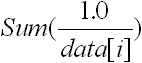This function takes the following tuple arguments:

• `double1`: data value.

Examples

Consider the query `qColtAggr48` in Example 11-84. Given the data stream `SColtAggrFunc` with schema `(c1 integer, c2 float, c3 double, c4 bigint)` in Example 11-85, the query returns the relation in Example 11-86.

Example 11-84 sumOfInversions Function Query

```<query id="qColtAggr48"><![CDATA[
select sumOfInversions(c3) from SColtAggrFunc
]]></query>
```

Example 11-85 sumOfInversions Function Stream Input

```Timestamp   Tuple
10        1, 0.5, 40.0, 8
1000        4, 0.7, 30.0, 6
1200        3, 0.89, 20.0, 12
2000        8, 0.4, 10.0, 4
h 8000
h 200000000
```

Example 11-86 sumOfInversions Function Relation Output

```Timestamp   Tuple Kind  Tuple
-9223372036854775808:+
10:       -
10:       +           Infinity
1000:       -           Infinity
1000:       +           Infinity
1200:       -           Infinity
1200:       +           Infinity
2000:       -           Infinity
2000:       +           Infinity
```

## sumOfLogarithms

Syntax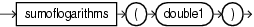Purpose

`sumOfLogarithms` is based on `cern.jet.stat.Descriptive.sumOfLogarithms(DoubleArrayList data, int from, int to)`. It returns the sum of logarithms of a data sequence (see Figure 11-16) as a `double`.

Figure 11-16 cern.jet.stat.Descriptive.sumOfLogarithms(DoubleArrayList data, int from, int to)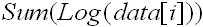This function takes the following tuple arguments:

• `double1`: data value.

Examples

Consider the query `qColtAggr49` in Example 11-87. Given the data stream `SColtAggrFunc` with schema `(c1 integer, c2 float, c3 double, c4 bigint)` in Example 11-88, the query returns the relation in Example 11-89.

Example 11-87 sumOfLogarithms Function Query

```<query id="qColtAggr49"><![CDATA[
select sumOfLogarithms(c3) from SColtAggrFunc
]]></query>
```

Example 11-88 sumOfLogarithms Function Stream Input

```Timestamp   Tuple
10        1, 0.5, 40.0, 8
1000        4, 0.7, 30.0, 6
1200        3, 0.89, 20.0, 12
2000        8, 0.4, 10.0, 4
h 8000
h 200000000
```

Example 11-89 sumOfLogarithms Function Relation Output

```Timestamp   Tuple Kind  Tuple
-9223372036854775808:+
10:       -
10:       +           -Infinity
1000:       -           -Infinity
1000:       +           -Infinity
1200:       -           -Infinity
1200:       +           -Infinity
2000:       -           -Infinity
2000:       +           -Infinity
```

## sumOfPowerDeviations

Syntax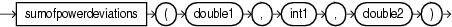Purpose

`sumOfPowerDeviations` is based on `cern.jet.stat.Descriptive.sumOfPowerDeviations(DoubleArrayList data, int k, double c)`. It returns sum of power deviations of a data sequence (see Figure 11-17) as a `double`.

Figure 11-17 cern.jet.stat.Descriptive.sumOfPowerDeviations(DoubleArrayList data, int k, double c)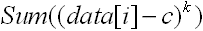This function is optimized for common parameters like `c == 0.0`, `k == -2 .. 4`, or both.

This function takes the following tuple arguments:

• `double1`: data value.

• `int1`: `k`.

• `double2`: `c`.

Examples

Consider the query `qColtAggr50` in Example 11-90. Given the data stream `SColtAggrFunc` with schema `(c1 integer, c2 float, c3 double, c4 bigint)` in Example 11-91, the query returns the relation in Example 11-92.

Example 11-90 sumOfPowerDeviations Function Query

```<query id="qColtAggr50"><![CDATA[
select sumOfPowerDeviations(c3, c1, c3) from SColtAggrFunc
]]></query>
```

Example 11-91 sumOfPowerDeviations Function Stream Input

```Timestamp   Tuple
10        1, 0.5, 40.0, 8
1000        4, 0.7, 30.0, 6
1200        3, 0.89, 20.0, 12
2000        8, 0.4, 10.0, 4
h 8000
h 200000000
```

Example 11-92 sumOfPowerDeviations Function Relation Output

```Timestamp   Tuple Kind  Tuple
-9223372036854775808:+
10:       -
10:       +           0.0
1000:       -           0.0
1000:       +           10000.0
1200:       -           10000.0
1200:       +           9000.0
2000:       -           9000.0
2000:       +           6.818E11
```

## sumOfPowers

Syntax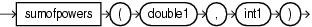Purpose

`sumOfPowers` is based on `cern.jet.stat.Descriptive.sumOfPowers(DoubleArrayList data, int k)`. It returns the sum of powers of a data sequence (see Figure 11-18) as a `double`.

Figure 11-18 cern.jet.stat.Descriptive.sumOfPowers(DoubleArrayList data, int k)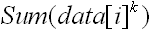This function takes the following tuple arguments:

• `double1`: data value.

• `int1`: `k`.

Examples

Consider the query `qColtAggr52` in Example 11-93. Given the data stream `SColtAggrFunc` with schema `(c1 integer, c2 float, c3 double, c4 bigint)` in Example 11-94, the query returns the relation in Example 11-95.

Example 11-93 sumOfPowers Function Query

```<query id="qColtAggr52"><![CDATA[
select sumOfPowers(c3, c1) from SColtAggrFunc
]]></query>
```

Example 11-94 sumOfPowers Function Stream Input

```Timestamp   Tuple
10        1, 0.5, 40.0, 8
1000        4, 0.7, 30.0, 6
1200        3, 0.89, 20.0, 12
2000        8, 0.4, 10.0, 4
h 8000
h 200000000
```

Example 11-95 sumOfPowers Function Relation Output

```Timestamp   Tuple Kind  Tuple
-9223372036854775808:+
10:       -
10:       +           40.0
1000:       -           40.0
1000:       +           3370000.0
1200:       -           3370000.0
1200:       +           99000.0
2000:       -           99000.0
2000:       +           7.2354E12
```

## sumOfSquaredDeviations

Syntax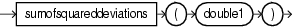Purpose

`sumOfSquaredDeviations` is based on `cern.jet.stat.Descriptive.sumOfSquaredDeviations(int size, double variance)`. It returns the sum of squared mean deviation of a data sequence (see Figure 11-19) as a `double`.

Figure 11-19 cern.jet.stat.Descriptive.sumOfSquaredDeviations(int size, double variance)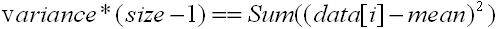This function takes the following tuple arguments:

• `double1`: data value.

Examples

Consider the query `qColtAggr53` in Example 11-96. Given the data stream `SColtAggrFunc` with schema `(c1 integer, c2 float, c3 double, c4 bigint)` in Example 11-97, the query returns the relation in Example 11-98.

Example 11-96 sumOfSquaredDeviations Function Query

```<query id="qColtAggr53"><![CDATA[
select sumOfSquaredDeviations(c3) from SColtAggrFunc
]]></query>
```

Example 11-97 sumOfSquaredDeviations Function Stream Input

```Timestamp   Tuple
10        1, 0.5, 40.0, 8
1000        4, 0.7, 30.0, 6
1200        3, 0.89, 20.0, 12
2000        8, 0.4, 10.0, 4
h 8000
h 200000000
```

Example 11-98 sumOfSquaredDeviations Function Relation Output

```Timestamp   Tuple Kind  Tuple
-9223372036854775808:+
10:       -
10:       +           0.0
1000:       -           0.0
1000:       +           25.0
1200:       -           25.0
1200:       +           133.33333333333334
2000:       -           133.33333333333334
2000:       +           375.0
```

## sumOfSquares

Syntax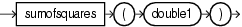Purpose

`sumOfSquares` is based on `cern.jet.stat.Descriptive.sumOfSquares(DoubleArrayList data)`. It returns the sum of squares of a data sequence (see Figure 11-20) as a `double`.

Figure 11-20 cern.jet.stat.Descriptive.sumOfSquares(DoubleArrayList data)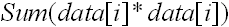This function takes the following tuple arguments:

• `double1`: data value.

Examples

Consider the query `qColtAggr54` in Example 11-99. Given the data stream `SColtAggrFunc` with schema `(c1 integer, c2 float, c3 double, c4 bigint)` in Example 11-100, the query returns the relation in Example 11-101.

Example 11-99 sumOfSquares Function Query

```<query id="qColtAggr54"><![CDATA[
select sumOfSquares(c3) from SColtAggrFunc
]]></query>
```

Example 11-100 sumOfSquares Function Stream Input

```Timestamp   Tuple
10        1, 0.5, 40.0, 8
1000        4, 0.7, 30.0, 6
1200        3, 0.89, 20.0, 12
2000        8, 0.4, 10.0, 4
h 8000
h 200000000
```

Example 11-101 sumOfSquares Function Relation Output

```Timestamp   Tuple Kind  Tuple
-9223372036854775808:+
10:       -
10:       +           1600.0
1000:       -           1600.0
1000:       +           2500.0
1200:       -           2500.0
1200:       +           2900.0
2000:       -           2900.0
2000:       +           3000.0
```

## trimmedMean

Syntax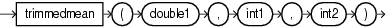Purpose

`trimmedMean` is based on `cern.jet.stat.Descriptive.trimmedMean(DoubleArrayList sortedData, double mean, int left, int right)`. It returns the trimmed mean of an ascending sorted data sequence as a `double`.

This function takes the following tuple arguments:

• `double1`: data value.

• `int1`: `left`.

• `int2`: `right`.

Examples

Consider the query `qColtAggr55` in Example 11-102. Given the data stream `SColtAggrFunc` with schema `(c1 integer, c2 float, c3 double, c4 bigint)` in Example 11-103, the query returns the relation in Example 11-104.

Example 11-102 trimmedMean Function Query

```<query id="qColtAggr55"><![CDATA[
select trimmedMean(c3, c1, c1) from SColtAggrFunc
]]></query>
```

Example 11-103 trimmedMean Function Stream Input

```Timestamp   Tuple
10        1, 0.5, 40.0, 8
1000        4, 0.7, 30.0, 6
1200        3, 0.89, 20.0, 12
2000        8, 0.4, 10.0, 4
h 8000
h 200000000
```

Example 11-104 trimmedMean Function Relation Output

```Timestamp   Tuple Kind  Tuple
-9223372036854775808:+
```

## variance

Syntax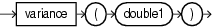Purpose

`variance` is based on `cern.jet.stat.Descriptive.variance(int size, double sum, double sumOfSquares)`. It returns the variance of a data sequence (see Figure 11-21) as a `double`.

Figure 11-21 cern.jet.stat.Descriptive.variance(int size, double sum, double sumOfSquares)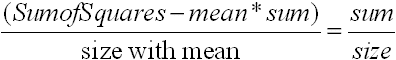This function takes the following tuple arguments:

• `double1`: data value.

Examples

Consider the query `qColtAggr57` in Example 11-105. Given the data stream `SColtAggrFunc` with schema `(c1 integer, c2 float, c3 double, c4 bigint)` in Example 11-106, the query returns the relation in Example 11-107.

Example 11-105 variance Function Query

```<query id="qColtAggr57"><![CDATA[
select variance(c3) from SColtAggrFunc
]]></query>
```

Example 11-106 variance Function Stream Input

```Timestamp   Tuple
10        1, 0.5, 40.0, 8
1000        4, 0.7, 30.0, 6
1200        3, 0.89, 20.0, 12
2000        8, 0.4, 10.0, 4
h 8000
h 200000000
```

Example 11-107 variance Function Relation Output

```Timestamp   Tuple Kind  Tuple
-9223372036854775808:+
10:       -
10:       +           0.0
1000:       -           0.0
1000:       +           25.0
1200:       -           25.0
1200:       +           66.66666666666667
2000:       -           66.66666666666667
2000:       +           125.0
```

## weightedMean

Syntax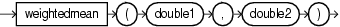Purpose

`weightedMean` is based on `cern.jet.stat.Descriptive.weightedMean(DoubleArrayList data, DoubleArrayList weights)`. It returns the weighted mean of a data sequence (see Figure 11-22) as a `double`.

Figure 11-22 cern.jet.stat.Descriptive.weightedMean(DoubleArrayList data, DoubleArrayList weights)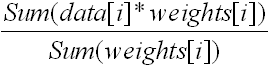This function takes the following tuple arguments:

• `double1`: data value.

• `double2`: weight value.

Examples

Consider the query `qColtAggr58` in Example 11-108. Given the data stream `SColtAggrFunc` with schema `(c1 integer, c2 float, c3 double, c4 bigint)` in Example 11-109, the query returns the relation in Example 11-110.

Example 11-108 weightedMean Function Query

```<query id="qColtAggr58"><![CDATA[
select weightedMean(c3, c3) from SColtAggrFunc
]]></query>
```

Example 11-109 weightedMean Function Stream Input

```Timestamp   Tuple
10        1, 0.5, 40.0, 8
1000        4, 0.7, 30.0, 6
1200        3, 0.89, 20.0, 12
2000        8, 0.4, 10.0, 4
h 8000
h 200000000
```

Example 11-110 weightedMean Function Relation Output

```Timestamp   Tuple Kind  Tuple
-9223372036854775808:+
10:       -
10:       +           40.0
1000:       -           40.0
1000:       +           35.714285714285715
1200:       -           35.714285714285715
1200:       +           32.22222222222222
2000:       -           32.22222222222222
2000:       +           30.0
```

## winsorizedMean

Syntax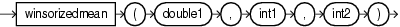Purpose

`winsorizedMean` is based on `cern.jet.stat.Descriptive.winsorizedMean(DoubleArrayList sortedData, double mean, int left, int right)`. It returns the winsorized mean of a sorted data sequence as a `double`.

This function takes the following tuple arguments:

• `double1`: data value.

• `int1`: `left`.

• `int2`: `right`.

Examples

Consider the query `qColtAggr60` in Example 11-111. Given the data stream `SColtAggrFunc` with schema `(c1 integer, c2 float, c3 double, c4 bigint)` in Example 11-112, the query returns the relation in Example 11-113.

Example 11-111 winsorizedMean Function Query

```<query id="qColtAggr60"><![CDATA[
select winsorizedMean(c3, c1, c1) from SColtAggrFunc
]]></query>
```

Example 11-112 winsorizedMean Function Stream Input

```Timestamp   Tuple
10        1, 0.5, 40.0, 8
1000        4, 0.7, 30.0, 6
1200        3, 0.89, 20.0, 12
2000        8, 0.4, 10.0, 4
h 8000
h 200000000
```

Example 11-113 winsorizedMean Function Relation Output

```Timestamp   Tuple Kind  Tuple
-9223372036854775808:+
```